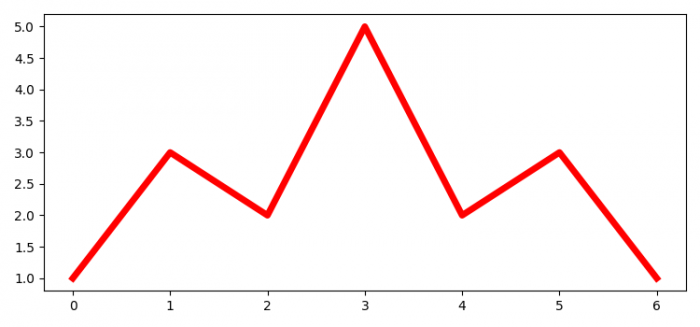# How to extract data from a Matplotlib plot?

To extract data from a plot in matplotlib, we can use get_xdata() and get_ydata() methods.

## Steps

• Set the figure size and adjust the padding between and around the subplots.
• Create y data points using numpy.
• Plot y data points with color=red and linewidth=5.
• Print a statment for data extraction.
• Use get_xdata() and get_ydata() methods to extract the data from the plot (step 3).
• Print x and y data (Step 5).
• To display the figure, use show() method.

## Example

import numpy as np
from matplotlib import pyplot as plt
plt.rcParams["figure.figsize"] = [7.50, 3.50]
plt.rcParams["figure.autolayout"] = True
y = np.array([1, 3, 2, 5, 2, 3, 1])
curve, = plt.plot(y, c='red', lw=5)
print("Extracting data from plot....")
xdata = curve.get_xdata()
ydata = curve.get_ydata()
print("X data points for the plot is: ", xdata)
print("Y data points for the plot is: ", ydata)
plt.show()

## Output

Extracting data from plot....
X data points for the plot is: [0. 1. 2. 3. 4. 5. 6.]
Y data points for the plot is: [1 3 2 5 2 3 1]